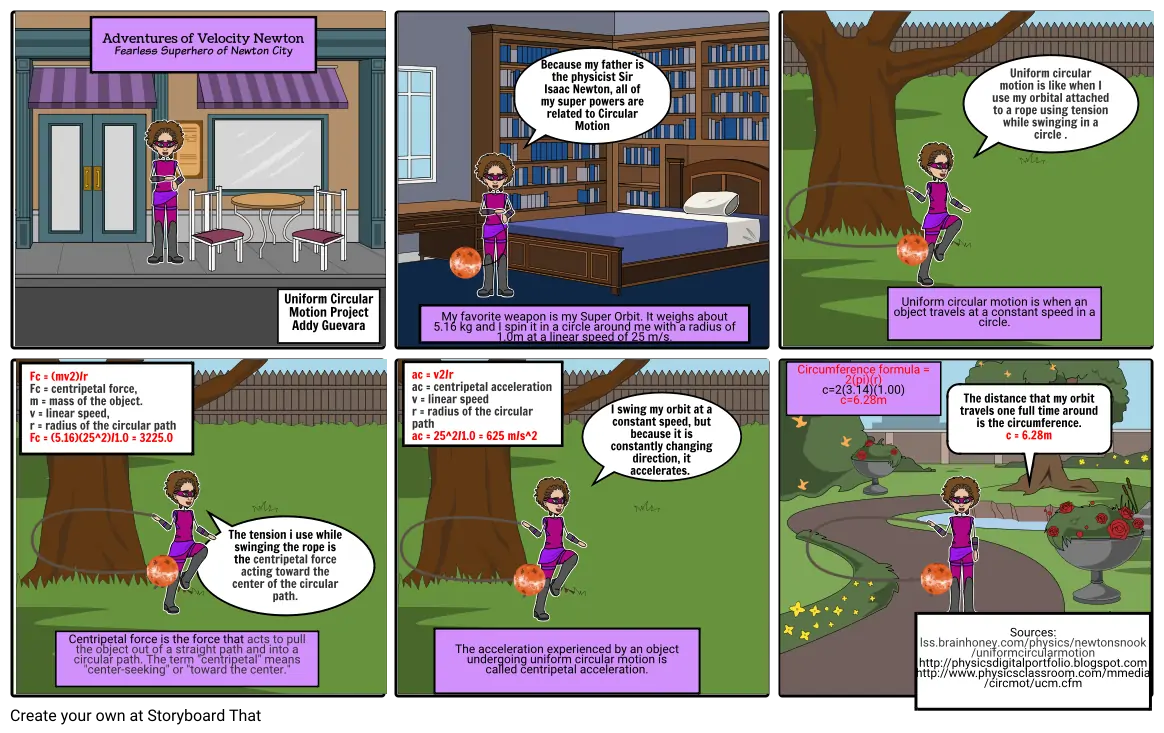# uniform circular motion#### Storyboard Text

• Adventures of Velocity Newton Fearless Superhero of Newton City
• ﻿Uniform Circular Motion Project Addy Guevara
• My favorite weapon is my Super Orbit. It weighs about 5.16 kg and I spin it in a circle around me with a radius of 1.0m at a linear speed of 25 m/s.﻿
• ﻿Because my father is the physicist Sir Isaac Newton,﻿ all of my super powers are related to Circular Motion
• Uniform circular motion is ﻿when an object travels at a constant speed in a circle.
• Uniform circular motion is like when I use my orbital attached to a rope using tension while swinging in a circle .﻿
• Fc = (mv2)/r Fc = centripetal force, m = mass of the object. v = linear speed, r = radius of the circular path Fc = (5.16)(25^2)/1.0 = 3225.0 ﻿
• Centripetal force is the force ﻿that acts to pull the object out of a straight path and into a circular path. The term "centripetal" means "center-seeking" or "toward the center."
• ﻿The tension i use while swinging the rope is the centripetal force acting toward the center of the circular path.
• ac = v2/r ac = centripetal acceleration v = linear speed r = radius of the circular path﻿ ac = 25^2/1.0 = 625 m/s^2
• The acceleration experienced by an object undergoing uniform circular motion is called centripetal acceleration.
• I swing my orbit at a constant speed, but because it is constantly changing direction, it accelerates. ﻿
• Circumference formula = 2(pi)(r) c=2(3.14)(1.00) c=6.28m﻿
• Sources: lss.brainhoney.com/physics/newtonsnook /uniformcircularmotion http://physicsdigitalportfolio.blogspot.com http://www.physicsclassroom.com/mmedia /circmot/ucm.cfm﻿
• ﻿The distance that my orbit travels one full time around is the circumference. c = 6.28m
##### Over 20 Million Storyboards Created